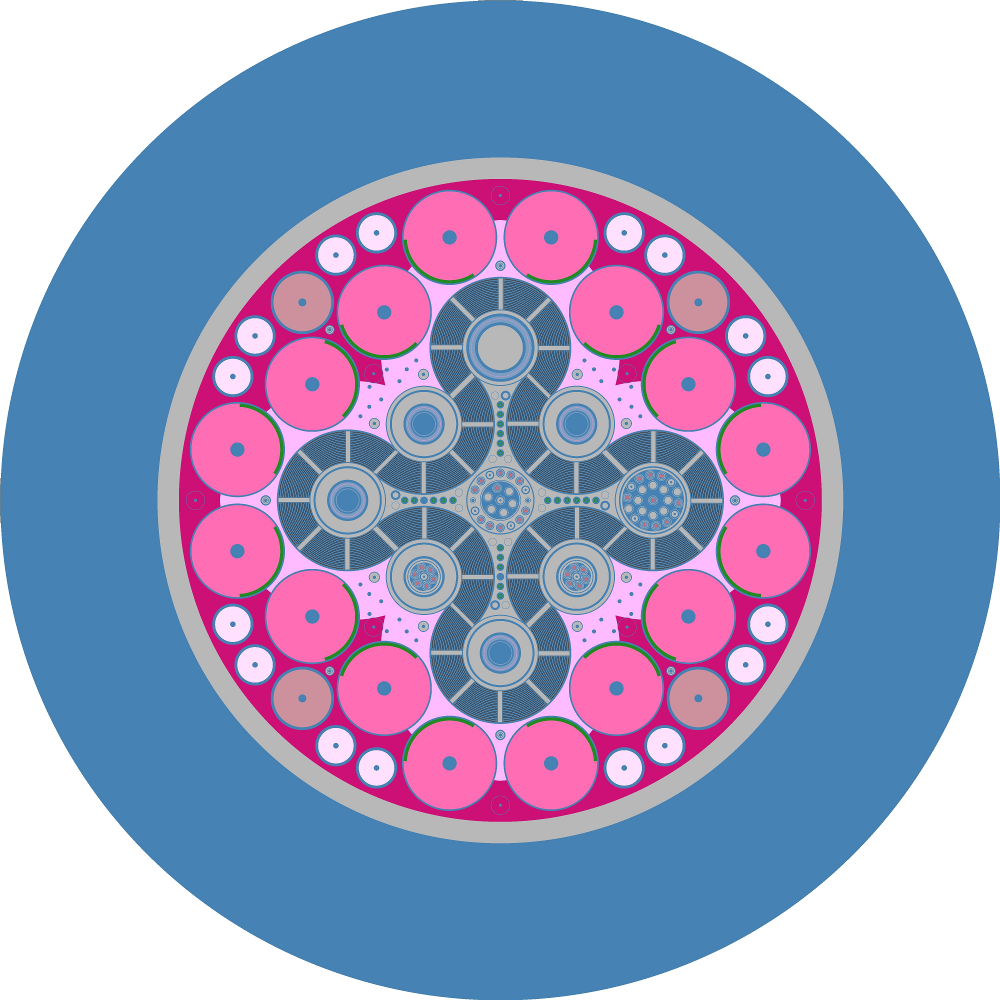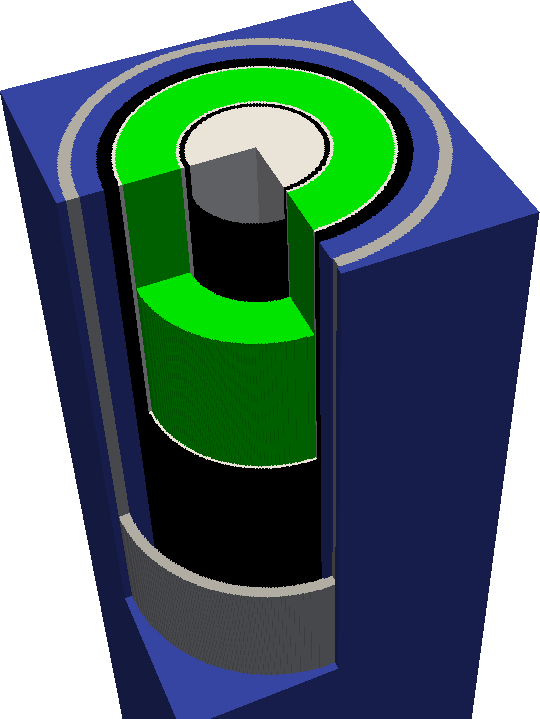# 9. Geometry Visualization¶

OpenMC is capable of producing two-dimensional slice plots of a geometry as well as three-dimensional voxel plots using the geometry plotting run mode. The geometry plotting mode relies on the presence of a plots.xml file that indicates what plots should be created. To create this file, one needs to create one or more openmc.Plot instances, add them to a openmc.Plots collection, and then use the Plots.export_to_xml method to write the plots.xml file.

## 9.1. Slice Plots¶By default, when an instance of openmc.Plot is created, it indicates that a 2D slice plot should be made. You can specify the origin of the plot (Plot.origin), the width of the plot in each direction (Plot.width), the number of pixels to use in each direction (Plot.pixels), and the basis directions for the plot. For example, to create a $$x$$ - $$z$$ plot centered at (5.0, 2.0, 3.0) with a width of (50., 50.) and 400x400 pixels:

plot = openmc.Plot()
plot.basis = 'xz'
plot.origin = (5.0, 2.0, 3.0)
plot.width = (50., 50.)
plot.pixels = (400, 400)


The color of each pixel is determined by placing a particle at the center of that pixel and using OpenMC’s internal find_cell routine (the same one used for particle tracking during simulation) to determine the cell and material at that location.

Note

In this example, pixels are 50/400=0.125 cm wide. Thus, this plot may miss any features smaller than 0.125 cm, since they could exist between pixel centers. More pixels can be used to resolve finer features but will result in larger files.

By default, a unique color will be assigned to each cell in the geometry. If you want your plot to be colored by material instead, change the Plot.color_by attribute:

plot.color_by = 'material'


If you don’t like the random colors assigned, you can also indicate that particular cells/materials should be given colors of your choosing:

plot.colors = {
water: 'blue',
}

# This is equivalent
plot.colors = {
water: (0, 0, 255),
}


Note that colors can be given as RGB tuples or by a string indicating a valid SVG color.

When you’re done creating your openmc.Plot instances, you need to then assign them to a openmc.Plots collection and export it to XML:

plots = openmc.Plots([plot1, plot2, plot3])
plots.export_to_xml()

# This is equivalent
plots = openmc.Plots()
plots.append(plot1)
plots += [plot2, plot3]
plots.export_to_xml()


To actually generate the plots, run the openmc.plot_geometry() function. Alternatively, run the openmc executable with the --plot command-line flag. When that has finished, you will have one or more .png files. Alternatively, if you’re working within a Jupyter Notebook or QtConsole, you can use the openmc.plot_inline() to run OpenMC in plotting mode and display the resulting plot within the notebook.

## 9.2. Voxel Plots¶The openmc.Plot class can also be told to generate a 3D voxel plot instead of a 2D slice plot. Simply change the Plot.type attribute to ‘voxel’. In this case, the Plot.width and Plot.pixels attributes should be three items long, e.g.:

vox_plot = openmc.Plot()
vox_plot.type = 'voxel'
vox_plot.width = (100., 100., 50.)
vox_plot.pixels = (400, 400, 200)


The voxel plot data is written to an HDF5 file. The voxel file can subsequently be converted into a standard mesh format that can be viewed in ParaView, VisIt, etc. This typically will compress the size of the file significantly. The provided openmc-voxel-to-vtk script can convert the HDF5 voxel file to VTK formats. Once processed into a standard 3D file format, colors and masks can be defined using the stored ID numbers to better explore the geometry. The process for doing this will depend on the 3D viewer, but should be straightforward.

Note

3D voxel plotting can be very computer intensive for the viewing program (Visit, ParaView, etc.) if the number of voxels is large (>10 million or so). Thus if you want an accurate picture that renders smoothly, consider using only one voxel in a certain direction.